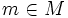# Restricted holonomy group

Let$M$ be a differential manifold,$E$ a vector bundle over$M$, and$\nabla$ a connection on$E$. The restricted holonomy group at$m \in M$ is defined as the subgroup of$GL(E_m)$ comprising all those linear transformations that occur as the holonomy of a loop that is homotopic to the constant loop.